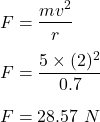Question

The diagram below shows a 5.0-kilogram bucket of water being swung in a horizontal circle of 0.70-meter radius
at a constant speed of 2.0 meters per second.
5.0-kg Bucket
0.70 m
The magnitude of the centripetal force on the bucket of water is approximately
1) 5.7 N
2) 14 N
3) 29 N
4) 200 N

1.Calantha

F = 29 N

Explanation:

We have,

Mass of the bucket of water is 5 kg

Radius of a horizontal circle is 0.7 m

Speed of the circle is 2 m/s

It is required to find the magnitude of centripetal force on the bucket of water. The formula used to find the magnitude of centripetal force is given by :or

F = 29 N

So, the centripetal force on the bucket of water is 29 N.